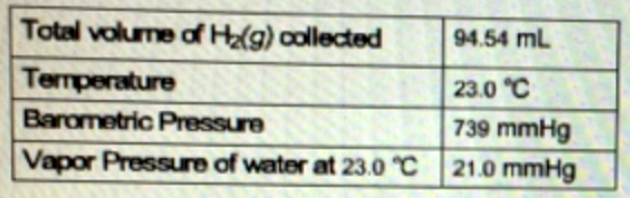# Problem: Hydrogen produced from a hydrolysis reaction was collected over water and the following data was compiled. Calculate the moles of hydrogen gas produced by the reaction.

###### FREE Expert Solution
93% (141 ratings)
###### FREE Expert Solution

We’re being asked to calculate the number of moles of the hydrogen gas produced from a hydrolysis reaction that is collected over water.

Hydrogen gas is the natural form of hydrogen and it is a diatomic molecule.

Hydrogen gas → H2(g)

We’re going to calculate the amount of hydrogen gas in moles using the ideal gas equation.

$\overline{){\mathbf{P}}{\mathbf{V}}{\mathbf{=}}{\mathbf{n}}{\mathbf{R}}{\mathbf{T}}}$

P = pressure, atm
V = volume, L
n = moles, mol
R = gas constant = 0.08206 (L·atm)/(mol·K)
T = temperature, K

Let's first calculate the Partial Pressure of hydrogen gas:

Given:            Total Pressure (Ptotal) = 739 mmHg

93% (141 ratings)###### Problem Details

Hydrogen produced from a hydrolysis reaction was collected over water and the following data was compiled.

Calculate the moles of hydrogen gas produced by the reaction.What scientific concept do you need to know in order to solve this problem?

Our tutors have indicated that to solve this problem you will need to apply the Collecting Gas Over Water concept. If you need more Collecting Gas Over Water practice, you can also practice Collecting Gas Over Water practice problems.

What is the difficulty of this problem?

Our tutors rated the difficulty ofHydrogen produced from a hydrolysis reaction was collected o...as medium difficulty.

How long does this problem take to solve?

Our expert Chemistry tutor, Dasha took 6 minutes and 23 seconds to solve this problem. You can follow their steps in the video explanation above.

What professor is this problem relevant for?

Based on our data, we think this problem is relevant for Professor Golde's class at PITT.